### Home > MC1 > Chapter 7 > Lesson 7.2.2 > Problem7-54

7-54.

Copy and complete each of the Diamond Problems below. The pattern used in the Diamond Problems is shown at right.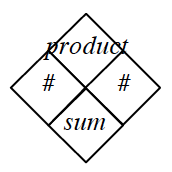1.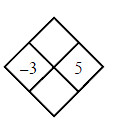For parts (a), (b), and (c), find the product and sum of the two numbers provided. Be sure to record your answers neatly!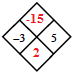The answers to part (a) are filled in above. Were you able to find the answers to parts (b) and (c)?

1.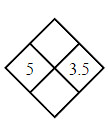1.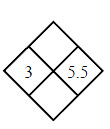1.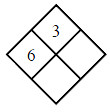You will know you are right when the product equals $3$.
Once you have found $y$, find the sum of $6$ and $y$ to complete the diamond!